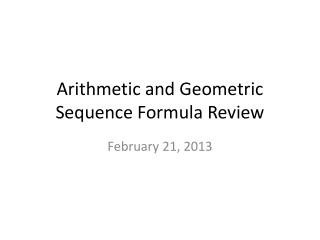Download PresentationArithmetic and Geometric Sequence Formula Review

Loading in 2 Seconds...

# Arithmetic and Geometric Sequence Formula Review - PowerPoint PPT Presentation

Arithmetic and Geometric Sequence Formula Review. February 21, 2013. Coordinate Algebra. Today’s Essential Question : How do I identify arithmetic and geometric sequences? How do I write recursive and explicit formulas for arithmetic and geometric sequences? Standard: MCC9-12.F.BF.2.I am the owner, or an agent authorized to act on behalf of the owner, of the copyrighted work described.
Download Presentation## Arithmetic and Geometric Sequence Formula Review

An Image/Link below is provided (as is) to download presentation

Download Policy: Content on the Website is provided to you AS IS for your information and personal use and may not be sold / licensed / shared on other websites without getting consent from its author.While downloading, if for some reason you are not able to download a presentation, the publisher may have deleted the file from their server.

- - - - - - - - - - - - - - - - - - - - - - - - - - E N D - - - - - - - - - - - - - - - - - - - - - - - - - -
Presentation Transcript
1. Arithmetic and Geometric Sequence Formula Review February 21, 2013

2. Coordinate Algebra Today’s Essential Question: How do I identify arithmetic and geometric sequences? How do I write recursive and explicit formulas for arithmetic and geometric sequences? Standard: MCC9-12.F.BF.2

3. Arithmetic & Geometric Sequences Recursive

4. Recursive Formula a formula used to find the next term of a sequence when the previous term is known

5. Recursive Formula for Arithmetic Sequence

6. Recursive Formula for Geometric Sequence

7. Find the next term and write the recursive rule.

8. Find the next term and write the recursive rule.

9. Write the explicit rule and the recursive rule. a1 = 15 and d = 5

10. Write the explicit rule and the recursive rule. a1 = 4 and r = .02